# 联邦学习：多任务思想与聚类联邦学习在联邦学习领域，许多传统机器学习已经讨论过的问题（甚至一些90年代和00年代的论文）都可以被再次被发明一次。比如我们会发现聚类联邦学习和多任务学习之间就有千丝万缕的联系。聚类联邦学习基本上都基于这样一个假设：虽然联邦学习中各节点的数据是Non-IID的，但是我们可以假定某些节点的数据可以归为一个聚类簇（簇内节点的分布近似IID）。实际上，这个思想让我们联想到高斯混合分布。高斯混合分布就假设每个节点的数据采样自高斯混合分布中的一个成分(对应一个簇)，而经典的高斯混合聚类就是要确定每个节点和簇的对应关系。

## 2. 多任务学习回顾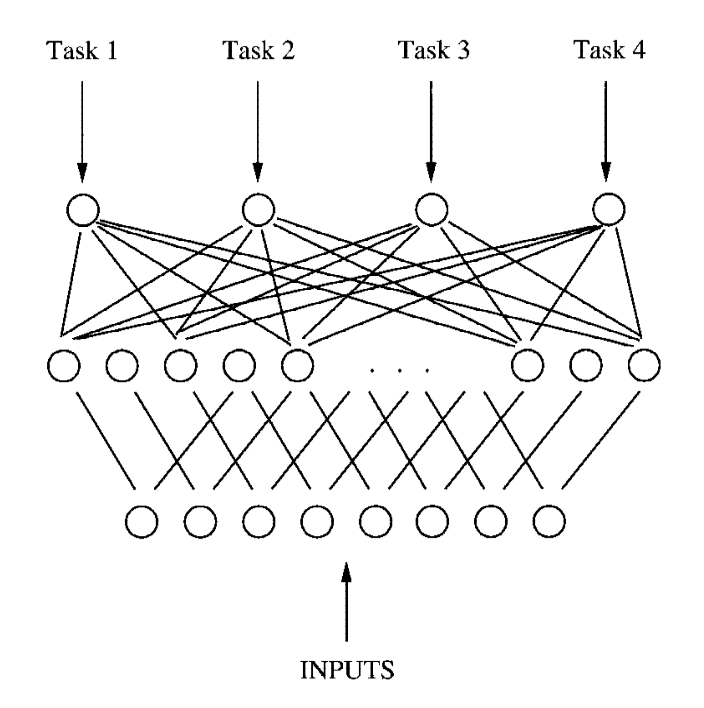$\bm{w}_t \sim \mathcal{N}(\bm{w}_t | \bm{u}, \mathbf{\Sigma}) \tag{1}$

$\bm{w}_t \sim \sum_{c=1}^C \alpha_c \mathcal{N} (\bm{w}_t | \bm{u}_c, \mathbf{\Sigma}_c) \tag{2}$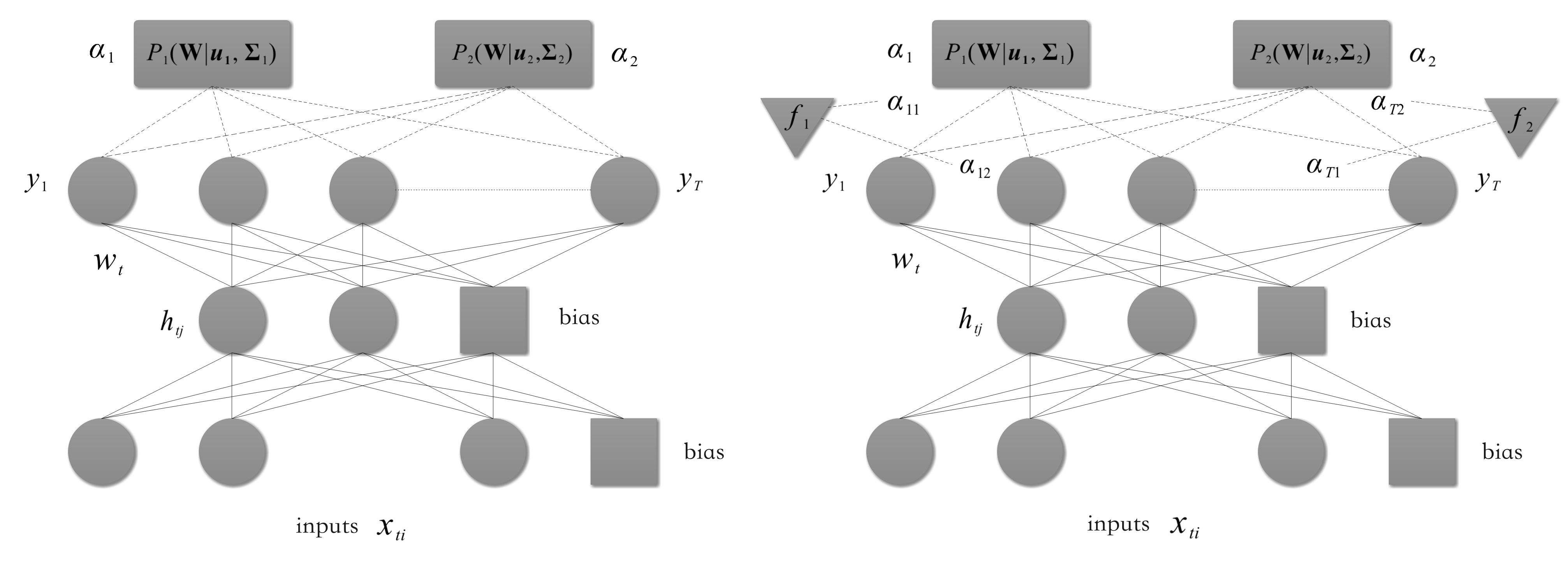## 3. 潜在聚类结构的的前置假设

（其实，很多论文这样创造聚类结构，并以此宣称自己的聚类算法优于非聚类算法如FedAvg，就好比医生故意把病人的腿打断了然后给他治病一样，也算是属于刻意去创造条件了，但或许科研就是这样吧）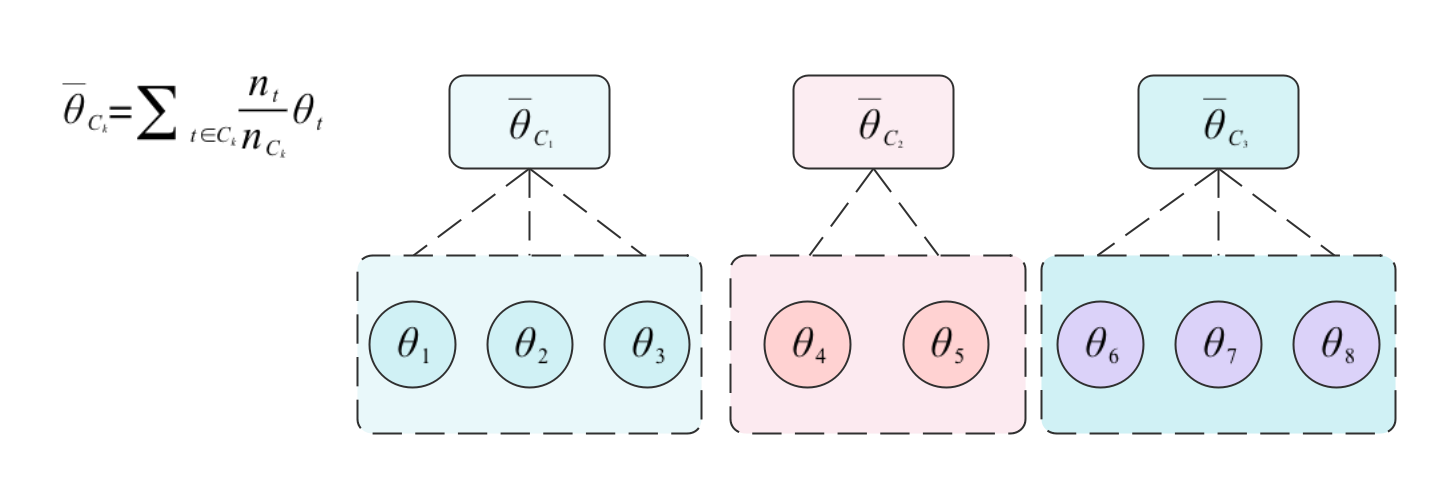## 4. 聚类联邦学习经典论文阅读

### 4.1 arXiv 2019:《Robust federated learning in a heterogeneous environment》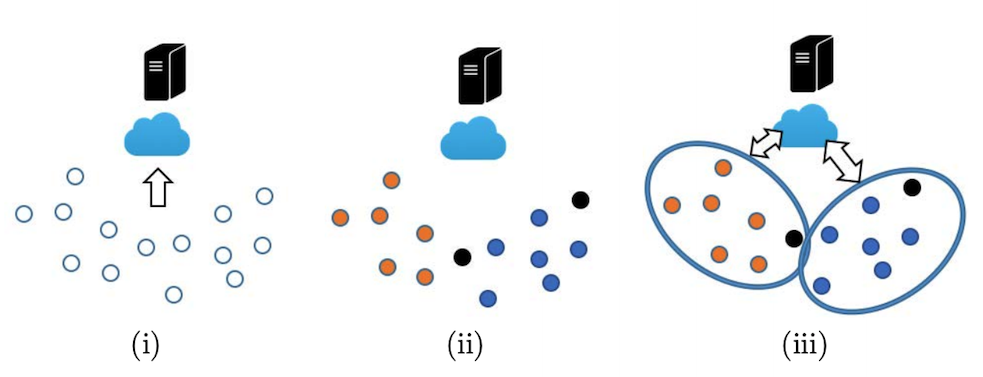### 4.2 TNNLS 2020:《Clustered Federated Learning: Model-Agnostic Distributed Multitask Optimization Under Privacy Constraints》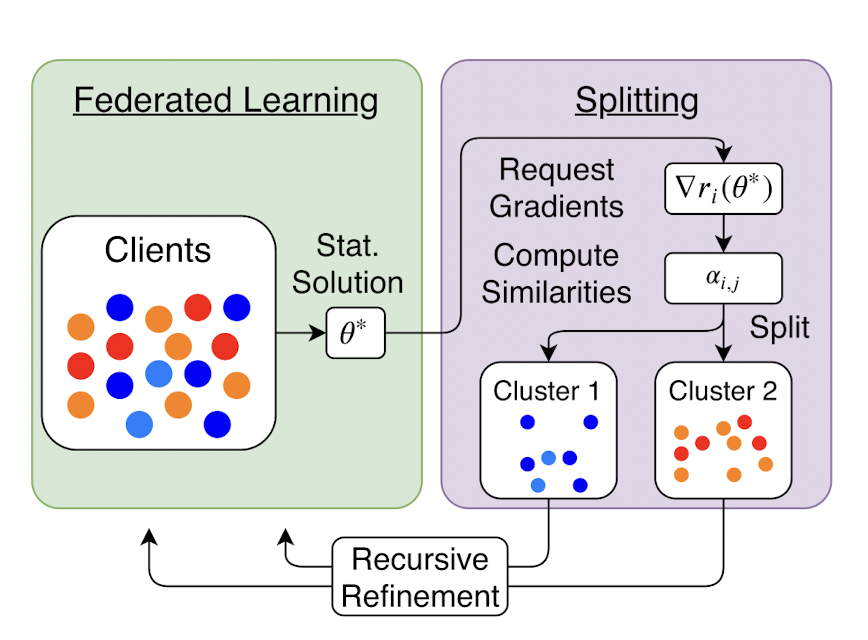(1) 第$$t$$个client节点执行

• 从server接收对应的簇参数$$g_k$$
• $$\theta_{old}=\theta_t=\theta_t + g_k$$
• 执行$$E$$个局部epoch的SGD：

$\theta_t = \theta_t – \eta \nabla \mathcal{l}(\theta_t; b)$

(此处将局部数据$$D_t$$划分为多个$$b$$)

• $$\widetilde{g}_t = \theta_t-\theta_{old}$$发往server。
• 重置$$\theta_t = \theta_{old}$$

(2) server节点执行

• $$T$$个client接收$$\widetilde{g}_1、\widetilde{g}_2,…\widetilde{g}_T$$

• 对每一个簇$$k$$，计算簇内平均参数变化：

$\hat{g}_{k} = \frac{1}{|S_k|}\sum_{t\in S_k}\widetilde{g}_t$

• 将每个簇的簇内变化$$\hat{g}_k$$发往对应的client。

• 根据不同节点参数变化量的余弦距离$$\alpha_{i,j}=\frac{\langle \widetilde{g}_i, \widetilde{g}_j\rangle}{||\widetilde{g}_i||||\widetilde{g}_j||}$$重新划分聚类簇。

### 4.3 NIPS 2020:《An Efficient Framework for Clustered Federated Learning》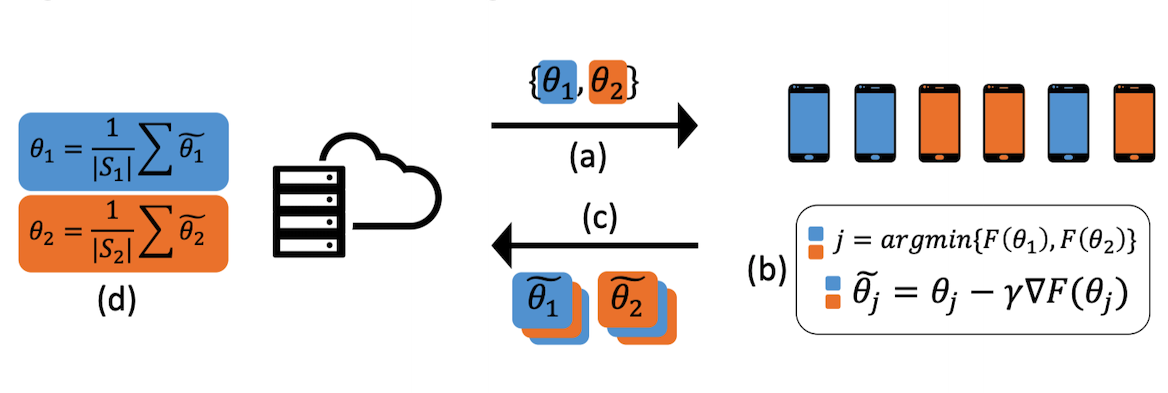(1) 第$$t$$个client节点执行

• 从server接收簇参数$$\theta_1,\theta_2,…\theta_{K}$$

• 估计其所属的簇：$$\hat{k}=\underset{k\in[K]}{\text{argmin }}\mathcal{l}(\theta_k;D_t)$$

• 对簇参数执行$$E$$个局部epoch的SGD：

$\theta_k = \theta_k – \eta \nabla \mathcal{l}(\theta_k; b)$

(此处将局部数据$$D_t$$划分为多个$$b$$)

• 将最终得到的簇参数做为$$\widetilde{\theta}_t$$，并和该client对应的簇划分一起发往server。

(2) server节点执行

• $$T$$个client接收$$\widetilde{\theta}_1、\widetilde{\theta}_2,…, \widetilde{\theta}_T$$和各client的簇划分情况

• 根据节点参数的平均值来更新簇参数：

$\hat{\theta}_k = \frac{1}{|S_k|} \sum_{t \in S_k}\widetilde{\theta}_t$

### 4.4 WWW 2021:《PFA: Privacy-preserving Federated Adaptation for Effective Model Personalization》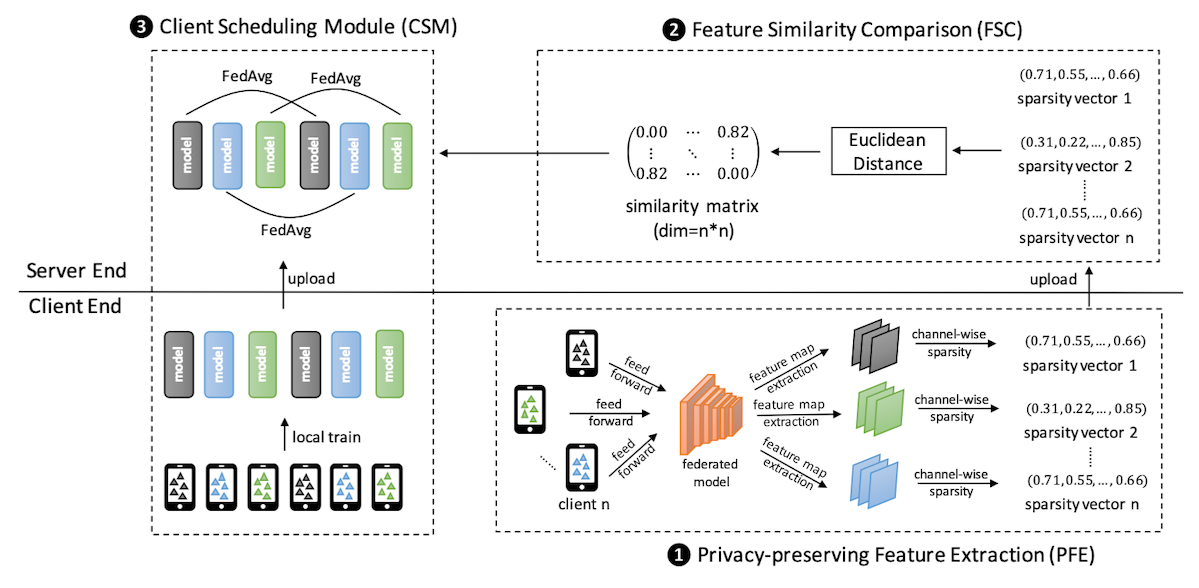### 4.5 INFOCOM 2021:《Resource-Efficient Federated Learning with Hierarchical Aggregation in Edge Computing》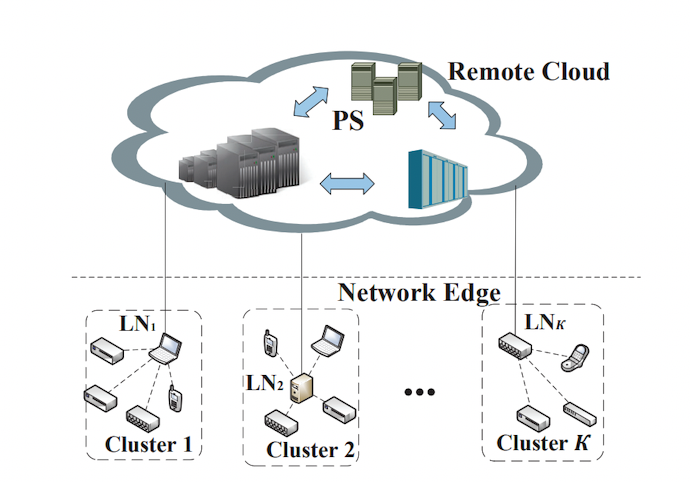(1) 第$$t$$个client节点执行

• $$LN_k$$接收参数$$\theta$$做为本地的$$\theta_t$$
• 执行$$E$$个局部epoch的SGD：

$\theta_t = \theta_t – \eta \nabla \mathcal{l}(\theta_t; b)$

(此处将局部数据$$D_t$$划分为多个$$b$$)

• 将新的参数$$\widetilde{\theta}_t$$发往所在簇的$$LN_k$$

(2) $$LN_k$$节点执行

• 从集群内的$$|S_k|$$个client接收参数$$\{\widetilde{\theta}_j\}(j\in S_k)$$

• 根据簇内节点参数的加权平均值来更新簇参数：

$\theta_k = \frac{\sum_{j \in S_k}{ |D_j|}\widetilde{\theta}_j}{\sum_{j\in S_k} |D_j|}$

• 将簇参数$$\theta_k$$发往PS。
• 从PS接收参数$$\theta$$并将其发往client节点。

(2) PS节点执行

• $$k$$$$LN_k$$节点接收$${\theta}_k$$（异步地）。

• 根据一阶指数平滑来更新参数：

$\hat{\theta} = (1-\alpha)\theta + \alpha \theta_k$

$$\quad\quad$$(在实际论文中$$\alpha$$是一个在迭代中变化的量，此处为了简化省略)

• 将新的参数$$\hat{\theta}$$发往$$LN_k$$

## 引用

•  Caruana R. Multitask learning[J]. Machine learning, 1997, 28(1): 41-75

•  Thrun S, O’Sullivan J. Discovering structure in multiple learning tasks: The TC algorithm[C]//ICML. 1996, 96: 489-497.

•  Bakker B J, Heskes T M. Task clustering and gating for bayesian multitask learning[J]. 2003.

•  Sattler F, Müller K R, Samek W. Clustered federated learning: Model-agnostic distributed multitask optimization under privacy constraints[J]. IEEE transactions on neural networks and learning systems, 2020, 32(8): 3710-3722.

•  McMahan B, Moore E, Ramage D, et al. Communication-efficient learning of deep networks from decentralized data[C]//Artificial intelligence and statistics. PMLR, 2017: 1273-1282.

•  Wang S, Roosta F, Xu P, et al. Giant: Globally improved approximate newton method for distributed optimization[J]. Advances in Neural Information Processing Systems, 2018, 31.

•  Ghosh A, Maity R K, Mazumdar A. Distributed newton can communicate less and resist byzantine workers[J]. Advances in Neural Information Processing Systems, 2020, 33: 18028-18038.

•  Ghosh A, Chung J, Yin D, et al. An efficient framework for clustered federated learning[J]. Advances in Neural Information Processing Systems, 2020, 33: 19586-19597.

•  Liu B, Guo Y, Chen X. PFA: Privacy-preserving Federated Adaptation for Effective Model Personalization[C]//Proceedings of the Web Conference 2021. 2021: 923-934.

•  Wang Z, Xu H, Liu J, et al. Resource-Efficient Federated Learning with Hierarchical Aggregation in Edge Computing[C]//IEEE INFOCOM 2021-IEEE Conference on Computer Communications. IEEE, 2021: 1-10.

•  Ghosh A, Hong J, Yin D, et al. Robust federated learning in a heterogeneous environment[J]. arXiv preprint arXiv:1906.06629, 2019.